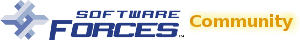# How to ... Standardize your Crystal Reports formula definitions?

When multiple reports share the same formula definition(s), i.e. you create a report for budgeting which contains a formula to calculate how many widgets are planned and called the formula definition `widget_planned`. You then needed a variation of this report, so you made a copy of it which means that the copy now also has a `widget_planned` formula.

Some time has gone by and you need to change the way the calculation works but you want to apply the change to the formula definition `widget_planned` in both reports to ensure they both reflect the change.

Using the the Formula Editor tool’s formula editing capabilities to make the change(s) to your formula definition(s) and then using the standardize formula capabilities is quick and easy.

1. Start by making change(s) to your formula definition as described in How to … Edit the formula definitions tutorial
2. Then in the Formula Editor tool page, for step 1, in the `Specify interaction to perform` tick on Standardize
3. Not every report you selected on the main portal grid may contain a formula. For the ones that do, in the left pane under step 2, they will be listed.
4. Navigate to the report which contains the source formula (the one that contains the formula definition that you’ll like to copy from) under the left pane under step 2, expand that report and select the source formula
a. only 1 source formula can be selected at a time
b. hover over the formula to see a preview of its formula definition
5. Now you need to specify the destination formula(s) (the one(s) that you want to copy to) in the right pane under step 3.
a. Here you can select multiple formula(s). If you select the entire report, all of it’s formulas will automatically be selected.
b. By selecting the destination formula definition(s), you are indicating that you want to overwrite their content with the content from the selected source formula.
c. hover over the formula to see a preview of its formula definition
6. Once you’re satisfied with your selections, click `Save Change(s)` button. This is disabled until a source is selected and at least 1 destination is selected.